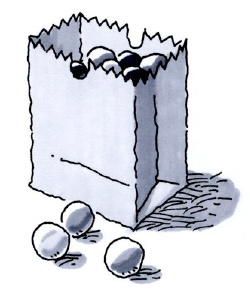### Home > CC2 > Chapter 1 > Lesson 1.2.2 > Problem1-70

1-70.

Imagine that you have a bag containing $10$ marbles of different colors. You have drawn a marble, recorded its color, and replaced it fifty times, with the following results: $9$ purple, $16$ orange, $6$ yellow, and $19$ green marbles. Make a prediction for how many marbles of each color are in the bag. Show all of your work or explain your reasoning.What is the experimental probability of drawing each color of marble?

Add up all of the draws.

$9+16+6+19=50$

Divide each number of draws by the total number to find the experimental probability.

$\frac{9}{50}=18$ percent purple, $\frac{16}{50}=32$ percent orange, $\frac{6}{50}=12$ percent Yellow, $\frac{19}{50}=38$ percent Green

Multiply by $10$ to find the number of each color of marble in the bag. Make sure to round to the nearest whole number.

$2$ purple, $3$ orange, $1$ yellow, $4$ green.